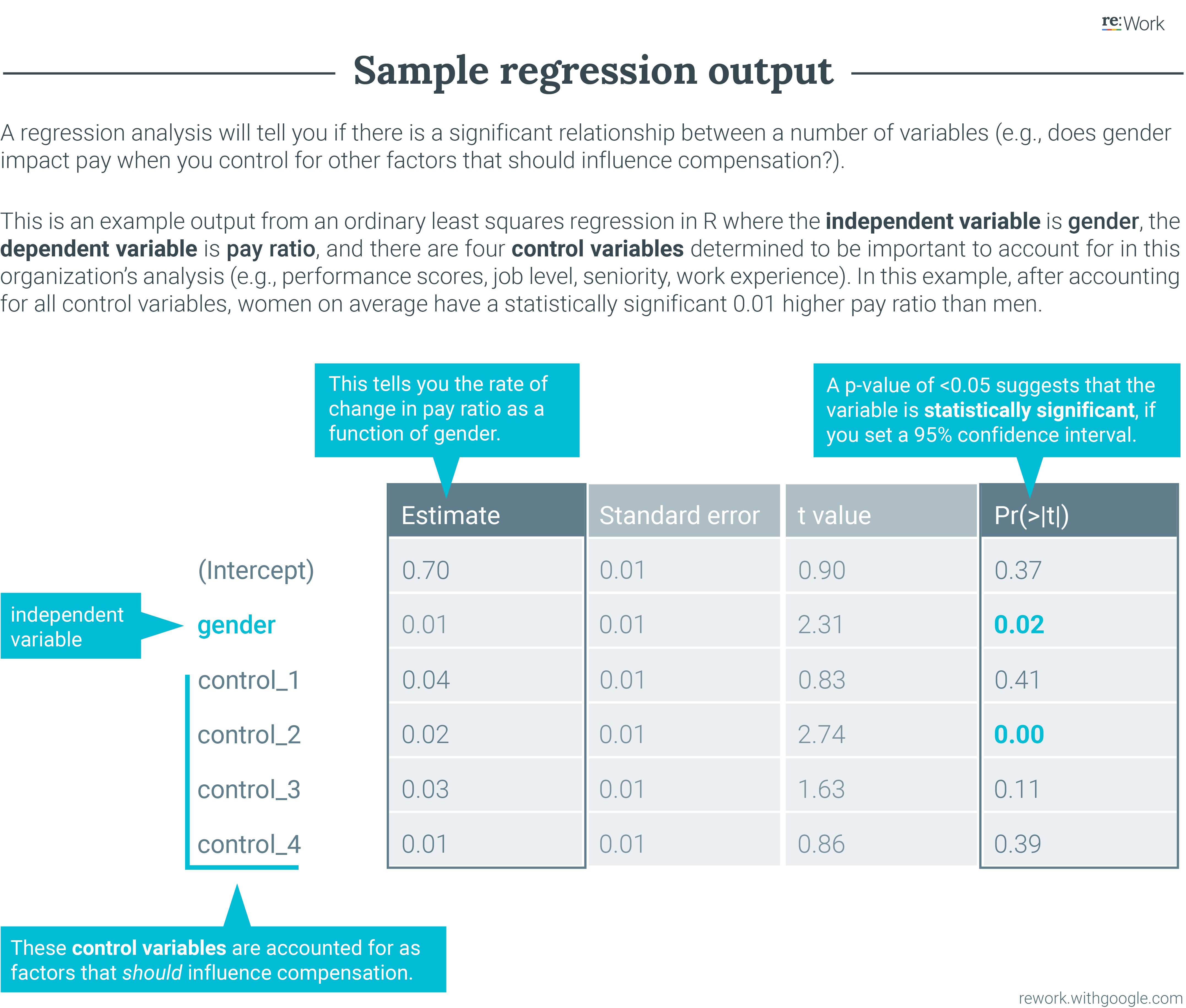Is this guide useful?

### buildTool: Analyze the data and look for variance

Once you have selected your variables, it’s time to begin the actual analysis. This requires someone with proficiency in statistics, including an understanding of standard deviation, variance, regression models, and the ability to interpret results.

1. Build and clean your data set. Good analyses rely on good data. Spot check your data to see if any data are missing or incorrect. If your data set is incomplete, understand why and determine if this might influence your results.
2. Identify substantially similar jobs. Pay equity analyses are most useful when comparing individuals doing similar work. You may have already grouped them when creating pay targets (e.g., entry level analysts in Sales may also be grouped with entry level analysts in Finance).
3. Check for multicollinearity, which occurs when two of the control variables are highly correlated and can result in drastic changes to your results. For example, tenure and job level could be highly correlated; the longer you’re at an organization the more likely you are to have moved up in level. Decide which variable is most important to control for and remove the other one from your regression analysis.
4. Run a regression analysis. The most common and rigorous method for conducting a thorough pay equity analysis is running a regression model. A regression will tell you if there is a significant relationship between a number of variables (e.g., analyzing if gender actually impacts pay when you control for other factors that should influence compensation). It helps you avoid conflating differences in compensation due to the independent variable (e.g., gender) and another variable that could legitimately explain differences in compensation (e.g., job level, which should influence compensation). Specifically, an ordinary least squares regression will allow you to analyze all the control variables at the same time (see how to run one in a spreadsheet or in R). Enter your control variables (e.g., job level) as step one in your regression. Next, add your independent variable (e.g., gender), and then the dependent variable (e.g., pay ratio).
5. Test for significance. Looking at your regression output, are there any statistically significant gaps between groups, as determined by a significance test? If so, conduct an effect size calculation to better understand the magnitude of any differences. Then, analyze which of the variables in your analysis account for any of those large gaps.
6. Check your work. Have a colleague review your methodology, your spreadsheet formulas or code, and your assumptions (e.g., how you define similar work). Review your descriptive statistics (e.g., averages, variance, correlations) to ensure that they make sense (e.g., does anyone have negative compensation? are there any outliers like someone earning \$1 per year?). Talk through your results with someone to see if they make logical sense.
7. Summarize your results. If your pay system is working well, at this stage you won’t see differences across gender or race/ethnicity. If you do find inequity, understand what’s causing it. Note any assumptions you made or variables you weren’t able to test and consider whether those could impact your results.Is this guide useful?